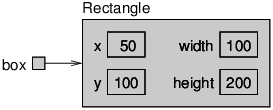Skip to main content

# 1.5: Mutable Objects

You can change the contents of an object by making an assignment to one of its attributes. For example, to “move” a rectangle without changing its size, you can modify the x and y values:

Rectangle box = new Rectangle(0, 0, 100, 200);
box.x = box.x + 50;
box.y = box.y + 100;


The result is shown in Figure 10.5.1.Figure $$\PageIndex{1}$$: State diagram showing updated attributes.

We can encapsulate this code in a method and generalize it to move the rectangle by any amount:

public static void moveRect(Rectangle box, int dx, int dy) {
box.x = box.x + dx;
box.y = box.y + dy;
}


The variables dx and dy indicate how far to move the rectangle in each direction. Invoking this method has the effect of modifying the Rectangle that is passed as an argument.

Rectangle box = new Rectangle(0, 0, 100, 200);
moveRect(box, 50, 100);
System.out.println(box);


Modifying objects by passing them as arguments to methods can be useful. But it can also make debugging more difficult, because it is not always clear which method invocations modify their arguments.

Java provides a number of methods that operate on Points and Rectangles. For example, translate has the same effect as moveRect, but instead of passing the rectangle as an argument, you use dot notation:

box.translate(50, 100);


This line invokes the translate method for the object that box refers to. As a result, the box object is updated directly.

This example is a good illustration of object-oriented programming. Rather than write methods like moveRect that modify one or more parameters, we apply methods to objects themselves using dot notation.